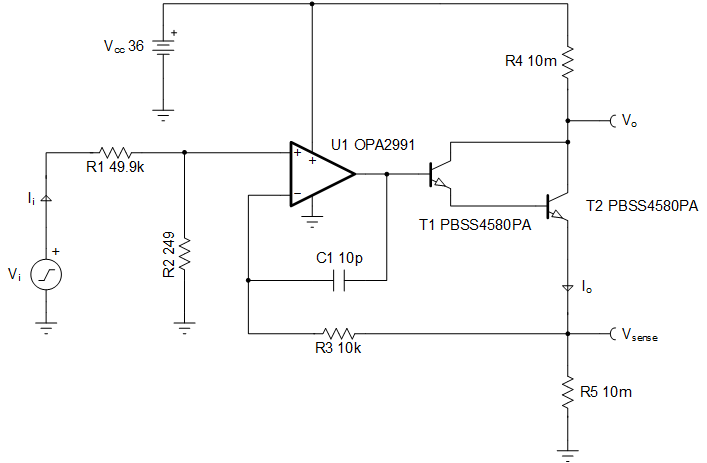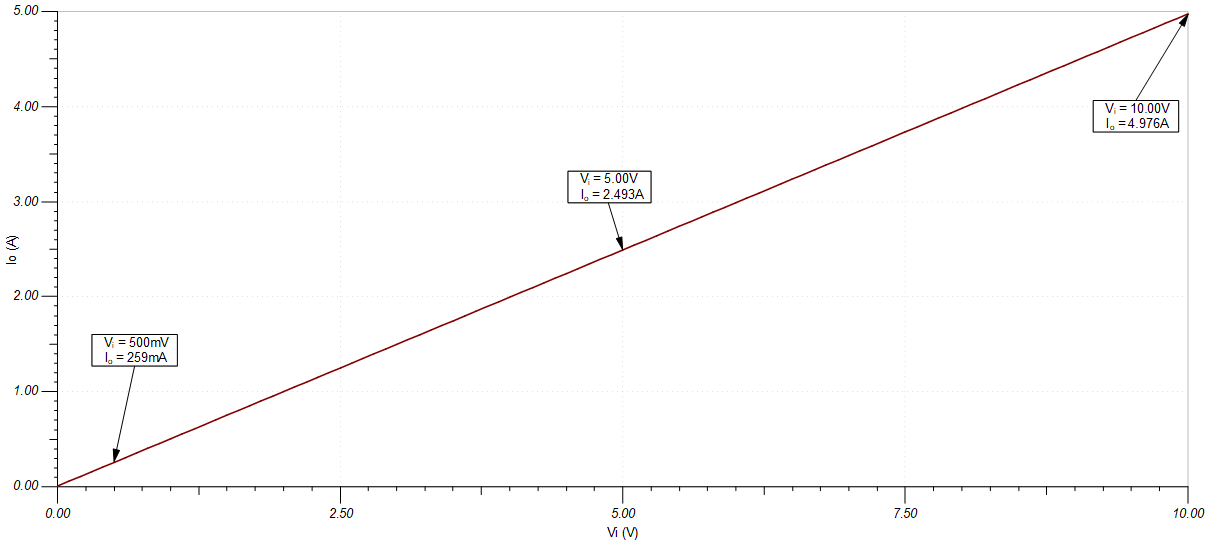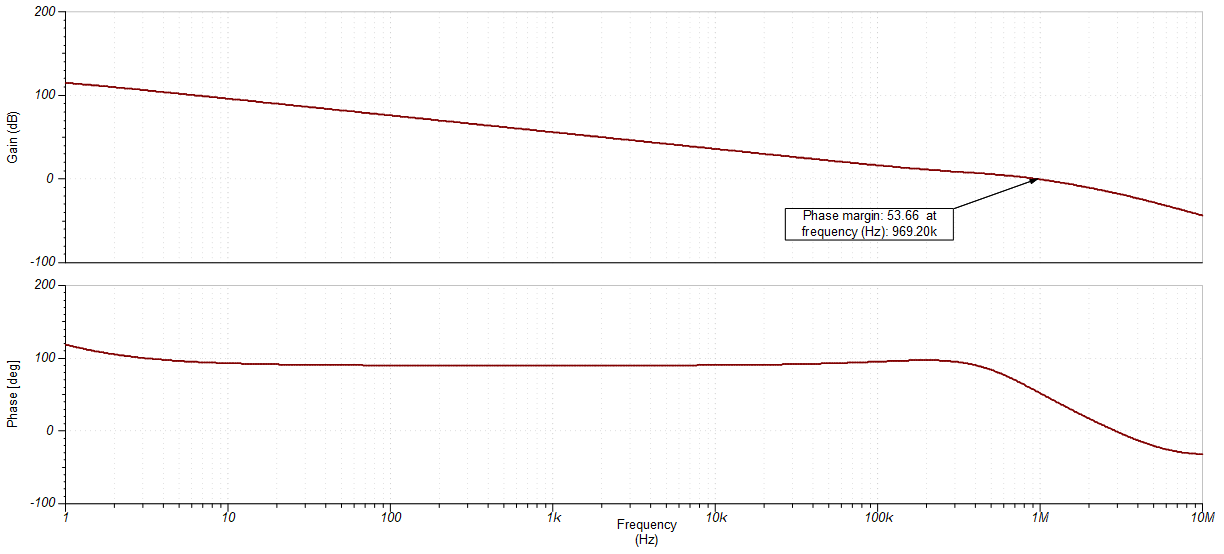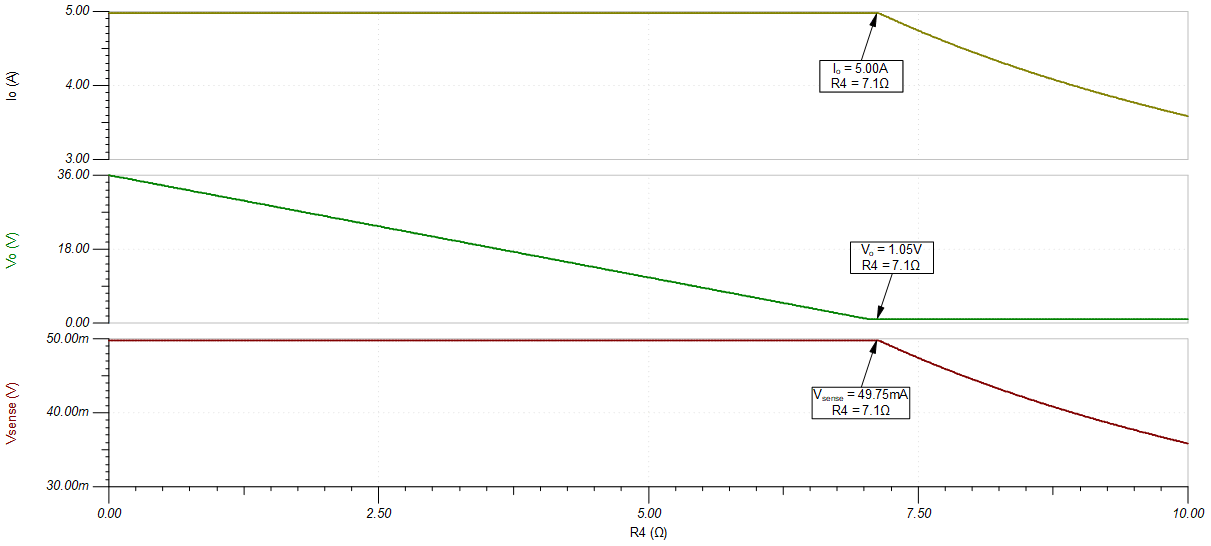SBOA326 May   2021

Design Goals

InputOutputSupply
ViMinViMaxIiMaxIoMinIoMaxPR5MaxVccVee
0V10V200µA0A5A0.25W36V0V

Design Description

This high-side voltage-to-current (V-I) converter delivers a well-regulated current to a load, R4. The circuit accepts an input voltage from 0V to 10V and converts it to an output current from 0A to 5A. The current is regulated by feeding the voltage across a low-side, current-sense resistor back to the op amp. The output Darlington pair allows for higher current gain than when using a single, discrete transistor.Design Notes

1. A resistor divider, formed by R1 and R2, is implemented at the input to limit the full-scale voltage at the non-inverting terminal of the amplifier and the output sense resistor (R5).
2. The high current gain of the Darlington pair reduces the demand on the output current of the op amp.
3. Smaller values of R4 and R5 lead to an increased load compliance voltage and a reduction in power dissipated in the full-scale, output state.
4. Feedback components R3 and C1 provide frequency compensation to ensure the stability of the circuit during transients. They also help reduce noise. R3 provides a DC feedback path directly at the current setting resistor, R5, and C1 provides a high-frequency feedback path that bypasses the NPN pair.
5. The input bias current will flow through R3, which will cause a DC error. Therefore, ensure that this error is minimal compared to the offset voltage of the op amp.
6. Select an op amp whose linear output voltage swing includes at least 2 ✕ Vbe+Vsense. The output voltage of the op amp will be greater than the voltage at the sense resistor by approximaly double the base-to-emitter voltage, Vbe.
7. Use the op amp in its linear operating region, specified under the AOL test conditions of the data sheet.
8. If needed, an isolation resistor may be placed between the high-frequency feedback path and the base of T1 for stability.

Design Steps

The transfer function of this circuit is provided in the following steps:

${I}_{o}={V}_{i}×\frac{{R}_{2}}{{R}_{5}×\left({R}_{1}+{R}_{2}\right)}$
1. Using the specifications for the maximum output power dissipation and the maximum output current, determine the maximum value of Vsense.
${V}_{R5\mathrm{Max}}={V}_{\mathrm{senseMax}}=\frac{{P}_{R5\mathrm{Max}}}{{I}_{\mathrm{oMax}}}=\frac{0.25W}{5A}=50\mathrm{mV}$
2. Calculate the sense resistance, R5.
${R}_{5}=\frac{{V}_{\mathrm{senseMax}}}{{I}_{\mathrm{oMax}}}=\frac{50mV}{5A}=10m\Omega$
3. Select values for R1 and R2 based on the maximum allowable input current, IiMax, and the desired VsenseMax voltage.
${R}_{1}=\frac{{V}_{\mathrm{senseMax}}}{{I}_{\mathrm{iMax}}}=\frac{50mV}{200\mu A}=250\Omega \approx 249\Omega \mathrm{\left(Standard Value\right)}$
${V}_{\mathrm{senseMax}}={V}_{\mathrm{iMax}}×\left(\frac{{R}_{2}}{{R}_{1}+{R}_{2}}\right)$
${R}_{2}=49.6k\Omega \approx 49.9k\Omega \left(Standard Value\right)$
4. See the Design References section  for the design procedure on how to properly size the compensation components, R3 and C1.

Design Simulations

DC Simulation ResultsLoop Stability Simulation Results

Loop gain phase is 53 degrees.Compliance Voltage Simulation ResultsDesign References

1. See Analog Engineer's Circuit Cookbooks for TI's comprehensive circuit library.
2. TI Precision Labs

Design Featured Op Amp

OPA2991
Vss2.7V to 40V
VinCMRail-to-rail
VoutRail-to-rail
Vos125µV
Iq560µA
Ib10pA
UGBW4.5MHz
SR21V/µs
#Channels1, 2, 4
www.ti.com/product/opa2991

Design Alternate Op Amp

OPA197
Vss4.5V to 36V
VinCMRail-to-rail
VoutRail-to-rail
Vos25µV
Iq1mA
Ib5pA
UGBW10MHz
SR20V/µs
#Channels1, 2, 4
www.ti.com/product/opa197